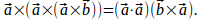## Proof Of Formula Using Vector Triple Product Formula

Theorem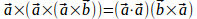(1)

Proof

The vector triple product formula is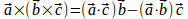(2)

In equation (1) write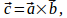then use (2) to give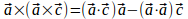Now use vec c = vec a times vec b to give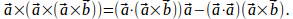vec a cdot (vec a times vec b )=0 so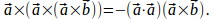Now use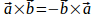to give